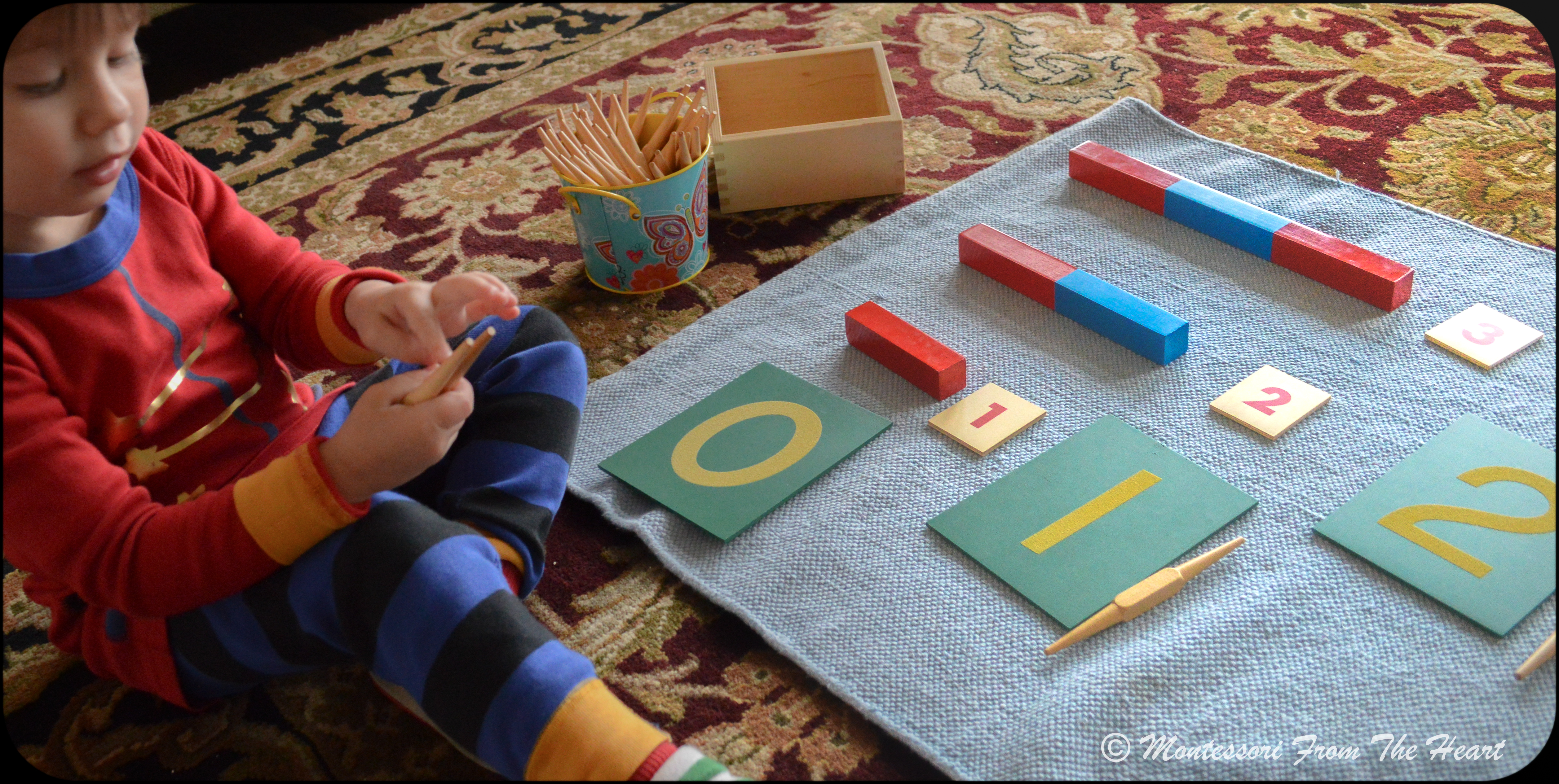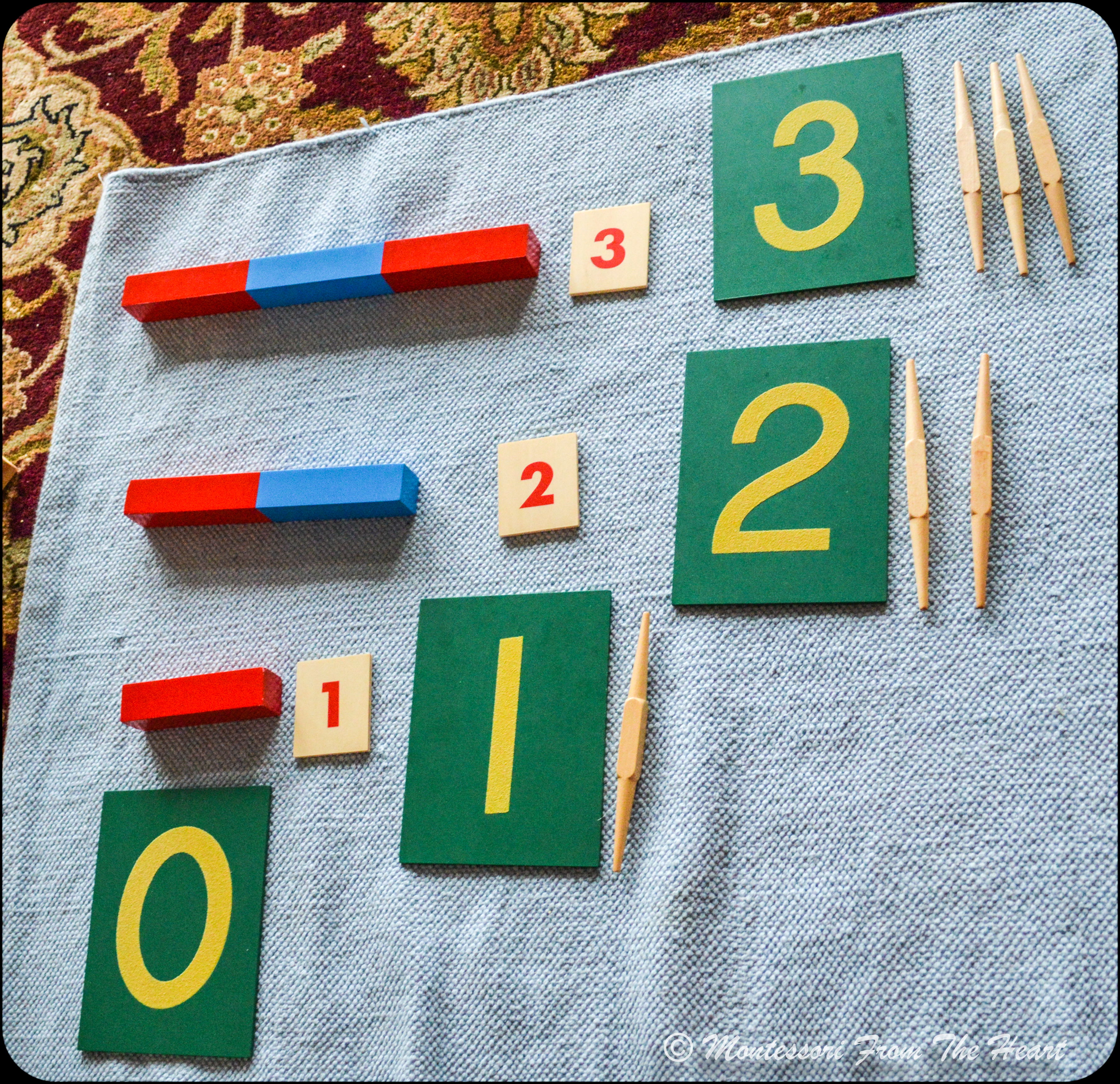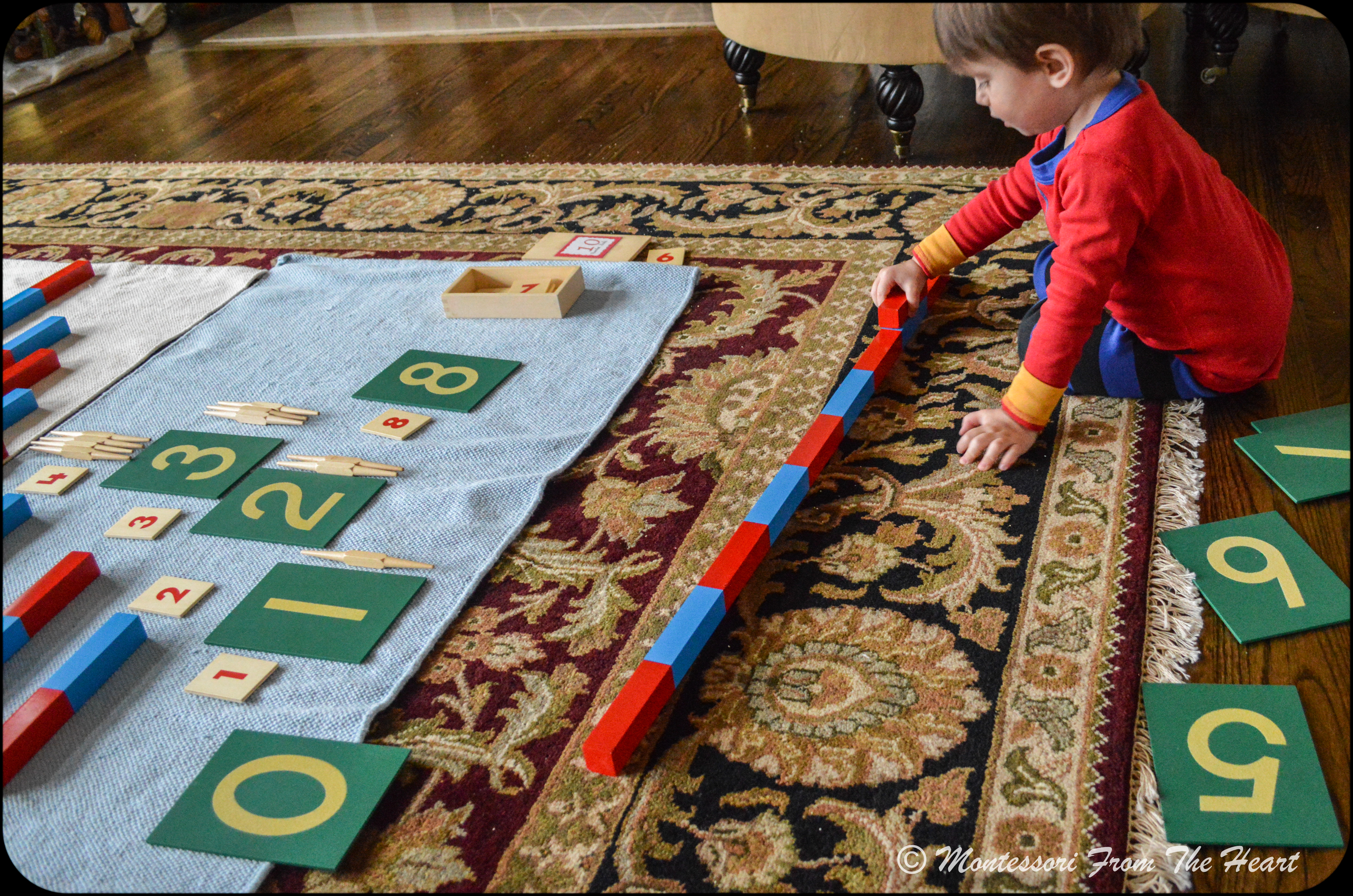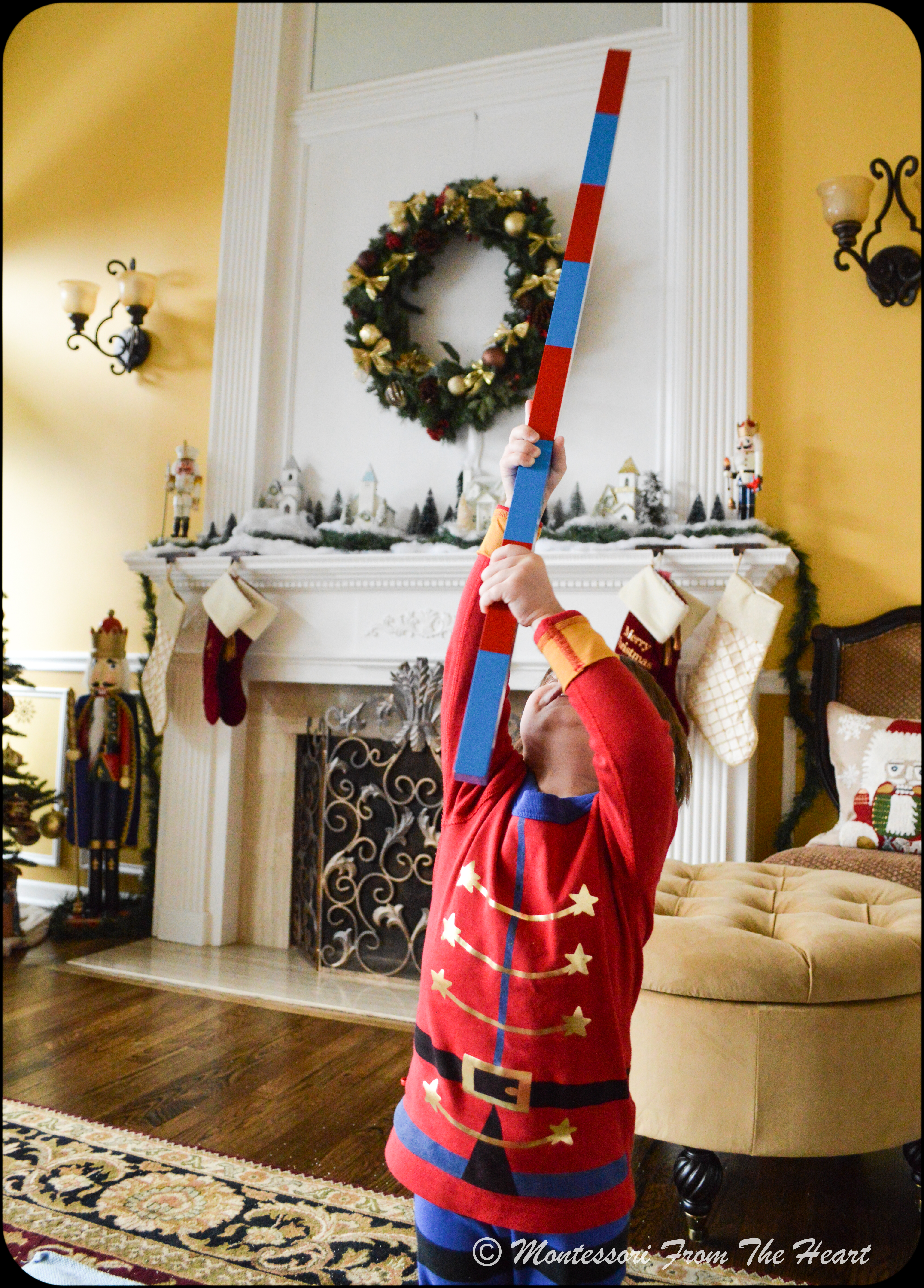Today, we are using a few traditional Montessori MATH materials to learn about quantity and numerals. In Montessori MATH curriculum, Numbers Rods are among the first math materials to introduce to a toddler at around two years of age (followed by Sandpaper Numbers and then by a Spindle Box).

However, simple PRACTICAL LIFE activities can be introduced even to a fifteen-month-old. Thereafter, you would naturally transition to SENSORIAL Activities in the following order: Knobbed Cylinders are usually introduced 1st; followed by a Pink Tower (2nd); followed by Brown/Broad stair (3rd); and then Red Rods (4th).

After the child is familiar with SENSORIAL Red rods, you would start with a MATH curriculum, by introducing the Numbers Rods (which are red and blue segmented). Today, we are using the Numbers Rods with number cards, spindles from the Spindle Box, and Sandpaper Numbers.

So the order of introducing Early Montessori Materials in a Nut Shell:

(I). PRACTICAL LIFE, starting at 15 months

(II). SENSORIAL materials

1. Knobbed Cylinders (2 yrs) – read a post here,
2. Pink Tower (2 yrs) – read a post here
4. Red Rods (2.5 yrs) – buy here,

(III). MATH materials

1. Numbers Rods (2.5 – 3 yrs) – buy here,
2. Sandpaper Numbers – read a post here,
3. Spindle Box – read a post here.

Adrian has been counting till ten in two languages since about at 27 months, however, I realized that he memorized the number sequence abstractly (like we recite a heart-learned poem) and that we needed to work on number recognition and quantity association, because when I would ask him to show me the numeral ("Where is number 2?"), he would get confused. So, it was time for Numbers Rods – learning that one is small and ten is big!

NUMBERS RODS (Montessori Numerical Rods)

Presentation: Toddlers need gross-motor stimulation, so position the Rods stand in the other room to offer a child an opportunity for movement. Ask a toddler to start bringing rods one by one starting with the smallest unit "1" (limiting to 3 rods per presentation.)

Present a 3🅿️🌠 Three Period Lesson:

1. (P1) “This is 1, this is 2, ….3” – introducing the quantity, actual rods (for example, for the "two rod" you can take a single unit rod and show 2 parts … we are just making 1 bigger)
2. (P2) “Will you show me 1? Will you show me 2? … 3? "
3. (P3) “What is this?

*If a child makes a mistake, rather than correcting, reiterate the lesson. For example, if, to your question: “Will you show me 2?" the child points to 1, you may proceed by saying: “This is 1, This is 2. Will you show me 2?”

For more on3🅿️🌠, read here a post on Montessori 3🅿️🌠 Three Period Lesson in Action (using First Phonetical Set: s m a t).

Today, we are trying an extension to this lesson by using also the number cards,  where you would associate Quantity (holding actual rods) to an abstract concept of Numbers and Numerals (symbols we chose to represent an actual quantity).  Here, the 1st Period would have a slight variation:

1.  (P1) This is 1 (lay an actual rod), and This says 1 (show a numeral card),
2. (P2) "Will you show me 1?"
3. (3) "What is this?"Adrian is exploring the quantity, as he applies abstract number memorization to practical implementation by counting 3 spindles.He then places spindles next to the "3" numeral – a symbol representing a quantity of actual rods he just counted.Adrian would then find the corresponding numeral amongst the Sandpaper Numbers trace it, and place next to its quantity.

For the 2nd Period,  Will you show me?" Adrian accurately points to all three numbers. However, with the 3rd Period: "What is this?" he doesn't always get it right.

With a 3🅿️🌠 Three Period Lesson, you would generally introduce three numbers at a time, so you would stop at number three. To instill the love of learning, we should end before the child's attention span starts to wane, or the child becomes tired, overwhelmed or overstimulated. The next time, you would introduce the next three numbers and so on. When the child is familiar with all numbers rods, you may also show that a larger number rod is made up of smaller units.By counting the "8-rod" using "1 unit-rod", he realizes that number 8 is comprised of 8 units!Having the muscular impression of length really solidifies the concept that 8 is big!

Numbers Rods are among the first Montessori math materials to introduce to a toddler at around two years of age to familiarize with the concept of quantity vs numeral. This traditional Montessori math material very concretely teaches a child that one is small and ten is big!Today, we are using animal figureens to make counting fun! We are placing an animal on each rod's segment.  One ~ giraffe. Two~ a lion and a lioness. Three ~ deer family. Four ~ gorilla family. Five ~bears: brown, black and a polar bear and their cubs. Six ~ family of cats: black panther, tiger, jaguar, cheetah, and a leopard.  Seven ~ horses.  Eight ~ farm family. Nine ~ birds. Ten ~ forest animals

p.s. I have been asked what is the difference between the Numbers Rods and Red Rods (buy here). The former is a math material (Numbers Rods), while the latter (Red Rods) is a sensorial material. Red rods is a material for teaching length: where the shortest piece is a unit of measurement for all the rest. As such, the second piece is double the first, the third is three times the first, etc., and, whilst the scale of length increases by ten centimeters for each piece, the other dimensions remain constant (i.e., the rods all have the same section). Presentation: show the child how to carry each rod to the mat by holding each rod at the top with one hand and at the bottom with the other in an upright position as to not bump into others (such gives the child a muscular impression of length). The rods should be randomly placed on the mat. Show the child how to build the rods starting with the shortest rod. Then, using your middle and index finger lightly trace along the entire length of the rod. Find the next longest rod. Bring it into position next to and above the previous rod and trace along its length again etc till the stair is entirely built. Pause and admire the stair and then mix up the rods and invite the child to try. Once the child has successfully built the stair, show him how to fit the shortest rod into each successive stair. Put them away starting with the longest first.